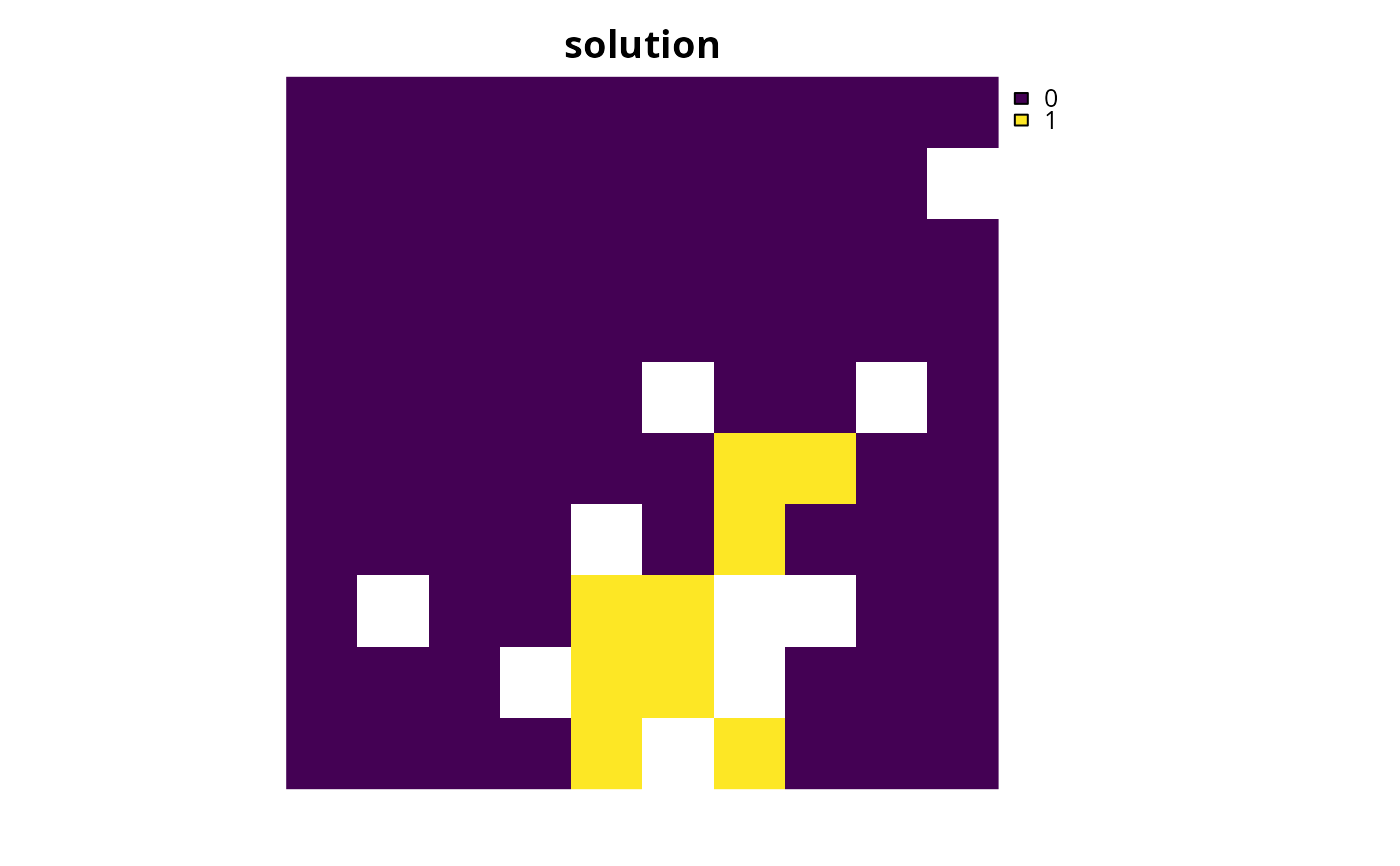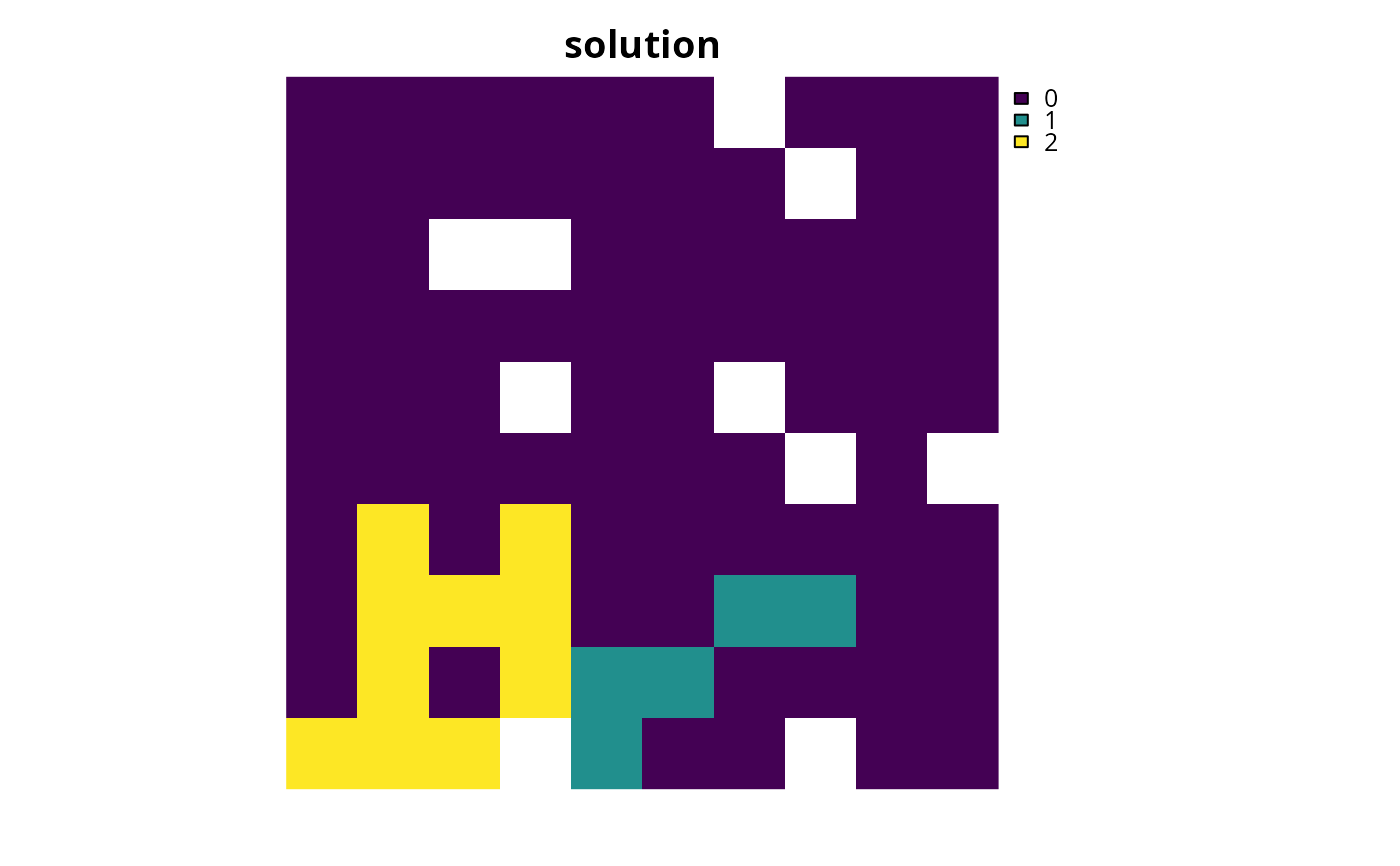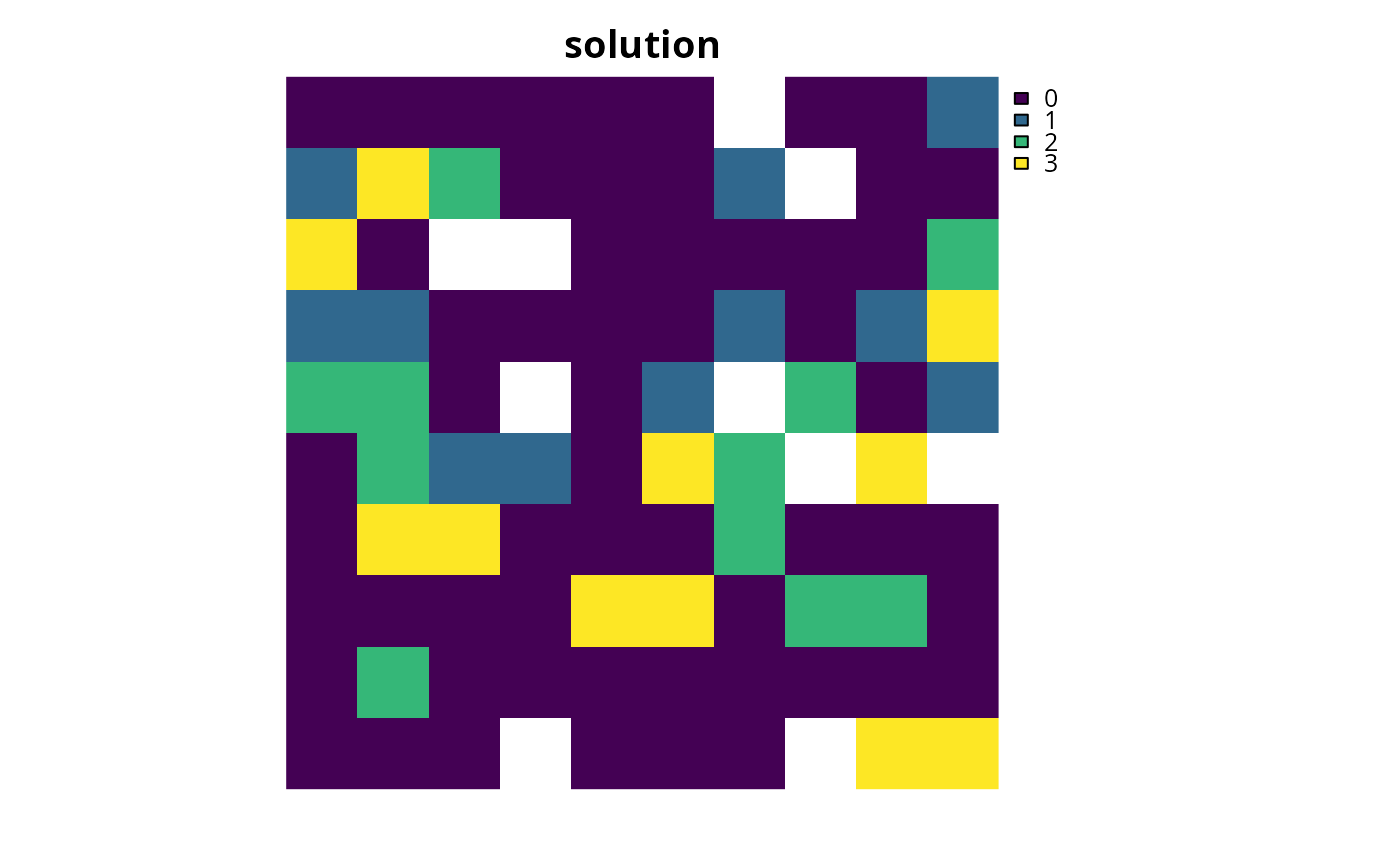Set the objective of a conservation planning problem() to minimize the overall shortfall for as many targets as possible while ensuring that the cost of the solution does not exceed a budget.

add_min_shortfall_objective(x, budget)

## Arguments

x

problem() (i.e., ConservationProblem) object.

budget

numeric value specifying the maximum expenditure of the prioritization. For problems with multiple zones, the argument to budget can be (i) a single numeric value to specify a single budget for the entire solution or (ii) a numeric vector to specify a separate budget for each management zone.

## Value

Object (i.e., ConservationProblem) with the objective added to it.

## Details

The minimum shortfall objective aims to find the set of planning units that minimize the overall (weighted sum) shortfall for the representation targets---that is, the fraction of each target that remains unmet---for as many features as possible while staying within a fixed budget (inspired by Table 1, equation IV, Arponen et al. 2005). Additionally, weights can be used to favor the representation of certain features over other features (see add_feature_weights().

## Mathematical formulation

This objective can be expressed mathematically for a set of planning units ($$I$$ indexed by $$i$$) and a set of features ($$J$$ indexed by $$j$$) as:

$$\mathit{Minimize} \space \sum_{j = 1}^{J} w_j \frac{y_j}{t_j} \\ \mathit{subject \space to} \\ \sum_{i = 1}^{I} x_i r_{ij} + y_j \geq t_j \forall j \in J \\ \sum_{i = 1}^{I} x_i c_i \leq B$$

Here, $$x_i$$ is the decisions variable (e.g., specifying whether planning unit $$i$$ has been selected (1) or not (0)), $$r_{ij}$$ is the amount of feature $$j$$ in planning unit $$i$$, $$t_j$$ is the representation target for feature $$j$$, $$y_j$$ denotes the representation shortfall for the target $$t_j$$ for feature $$j$$, and $$w_j$$ is the weight for feature $$j$$ (defaults to 1 for all features; see add_feature_weights() to specify weights). Additionally, $$B$$ is the budget allocated for the solution, $$c_i$$ is the cost of planning unit $$i$$. Note that $$y_j$$ is a continuous variable bounded between zero and infinity, and denotes the shortfall for target $$j$$.

See objectives for an overview of all functions for adding objectives. Also, see targets for an overview of all functions for adding targets, and add_feature_weights() to specify weights for different features.

Other objectives: add_max_cover_objective(), add_max_features_objective(), add_max_phylo_div_objective(), add_max_phylo_end_objective(), add_max_utility_objective(), add_min_largest_shortfall_objective(), add_min_set_objective()

## Examples

# load data
data(sim_pu_raster, sim_pu_zones_stack, sim_features, sim_features_zones)

# create problem with minimum shortfall objective
p1 <- problem(sim_pu_raster, sim_features) %>%
# \dontrun{
# solve problem
s1 <- solve(p1)

# plot solution
plot(s1, main = "solution", axes = FALSE, box = FALSE)# }

# create multi-zone problem with minimum shortfall objective,
# with 10% representation targets for each feature, and set
# a budget such that the total maximum expenditure in all zones
# cannot exceed 3000
p2 <- problem(sim_pu_zones_stack, sim_features_zones) %>%
add_relative_targets(matrix(0.1, ncol = 3, nrow = 5)) %>%
# \dontrun{
# solve problem
s2 <- solve(p2)

# plot solution
plot(category_layer(s2), main = "solution", axes = FALSE, box = FALSE)# }
# create multi-zone problem with minimum shortfall objective,
# with 10% representation targets for each feature, and set
# separate budgets for each management zone
p3 <- problem(sim_pu_zones_stack, sim_features_zones) %>%